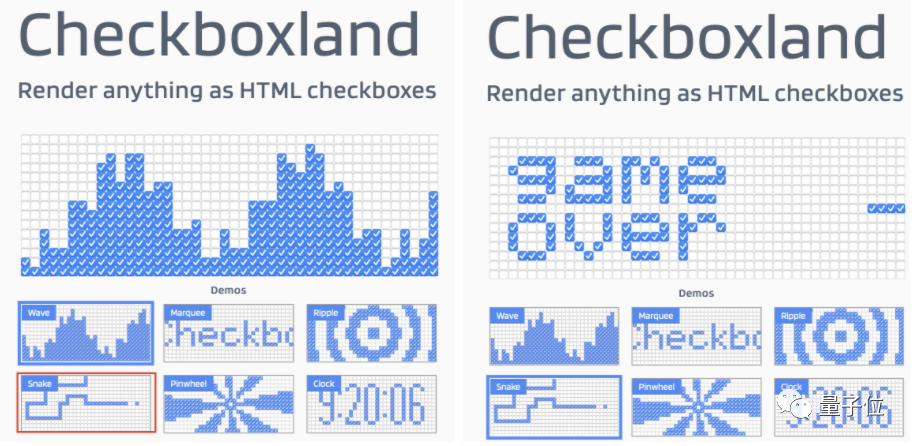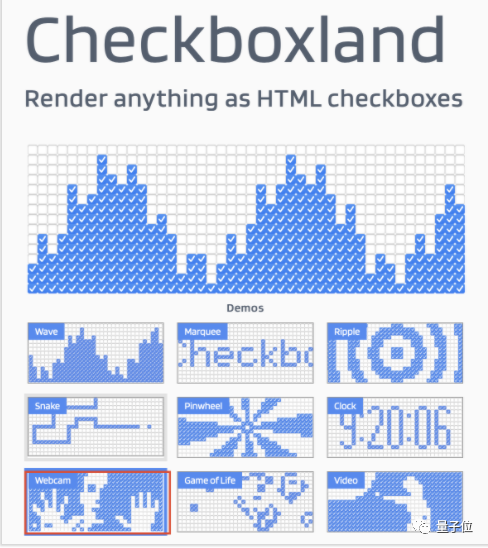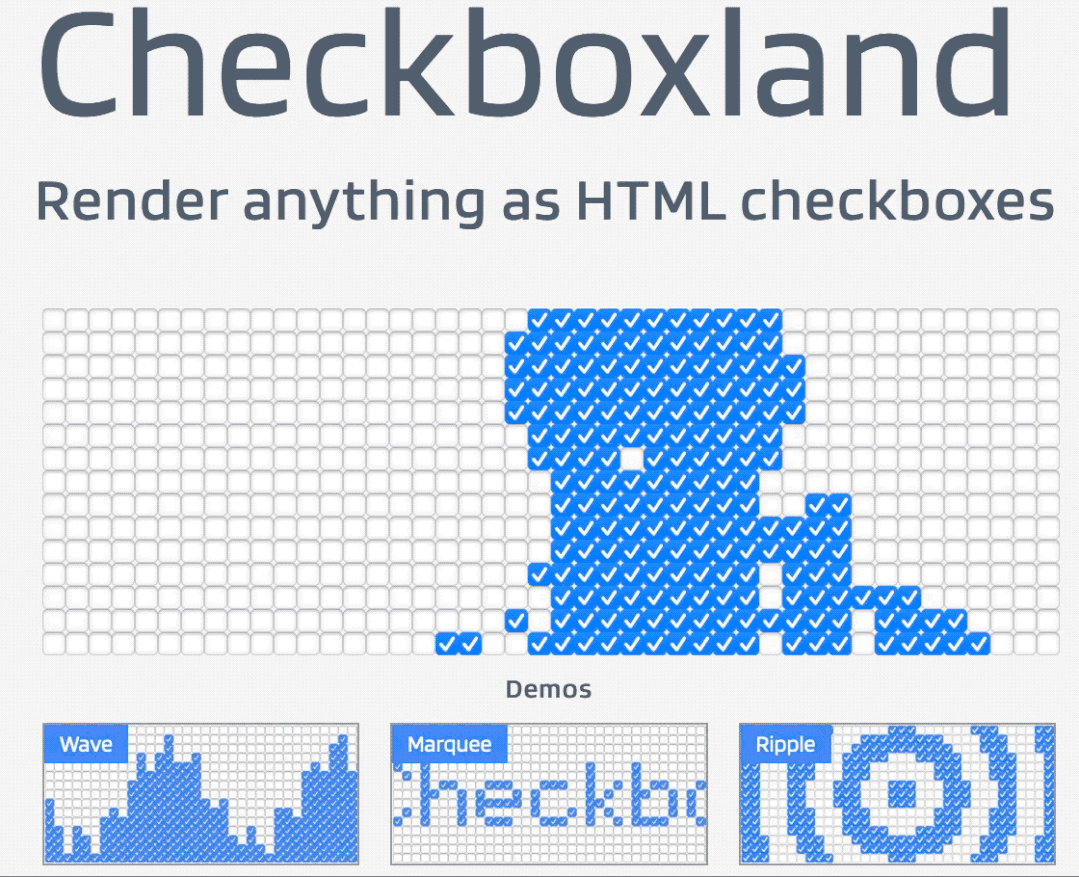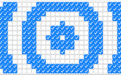# 前端小哥玩HTML复选框上瘾 能画logo做视频 还开源成JS库

2021年10月24日 17:50:41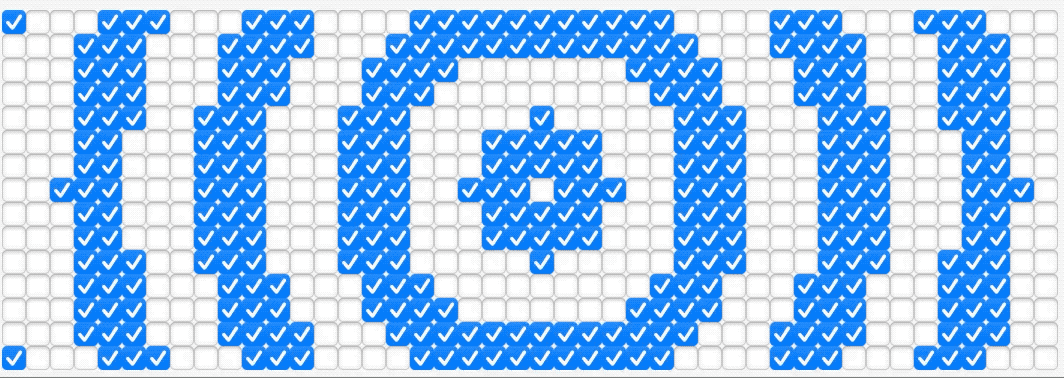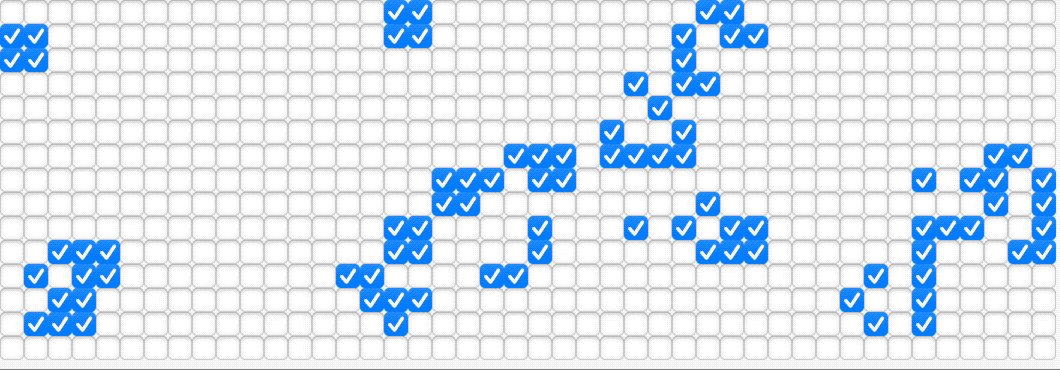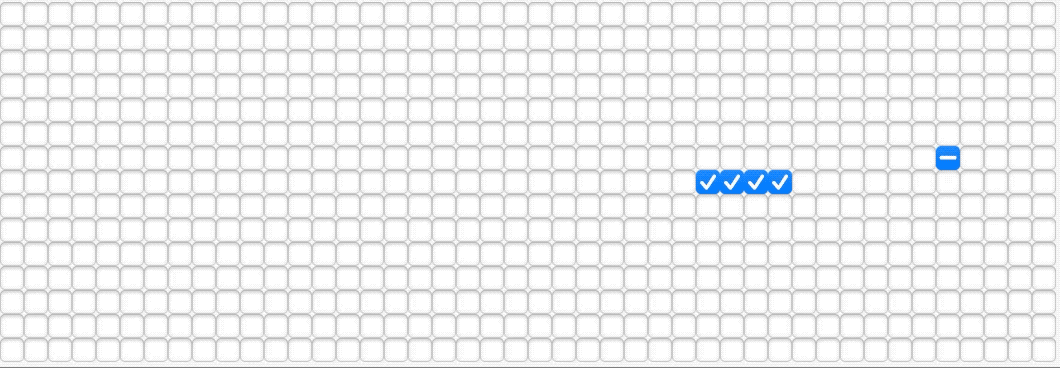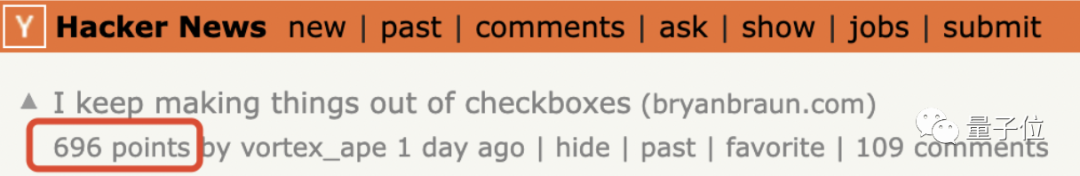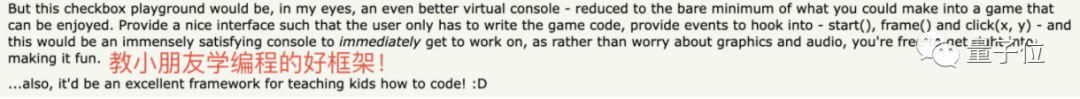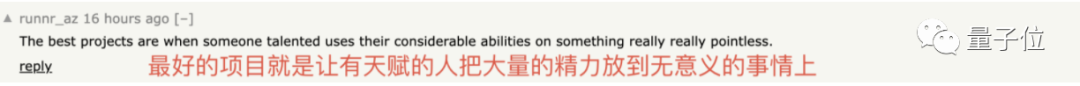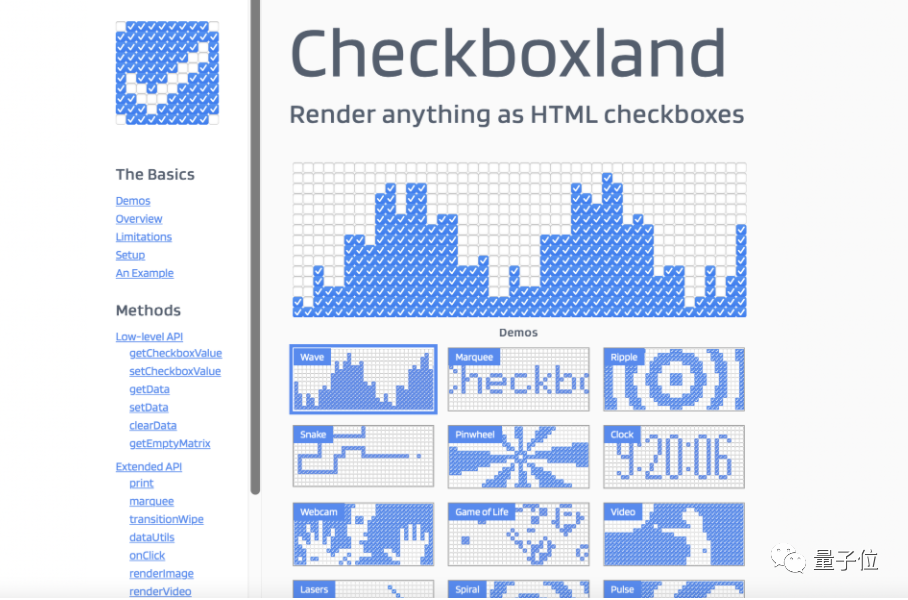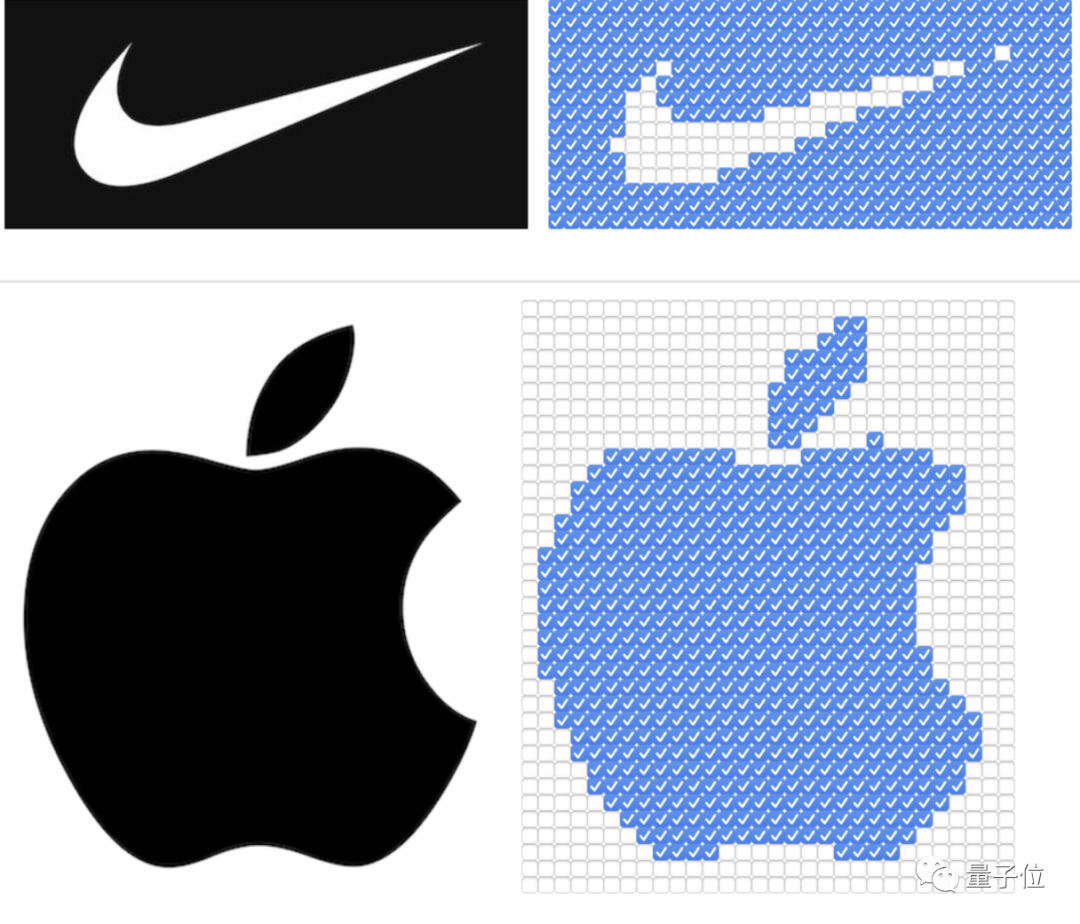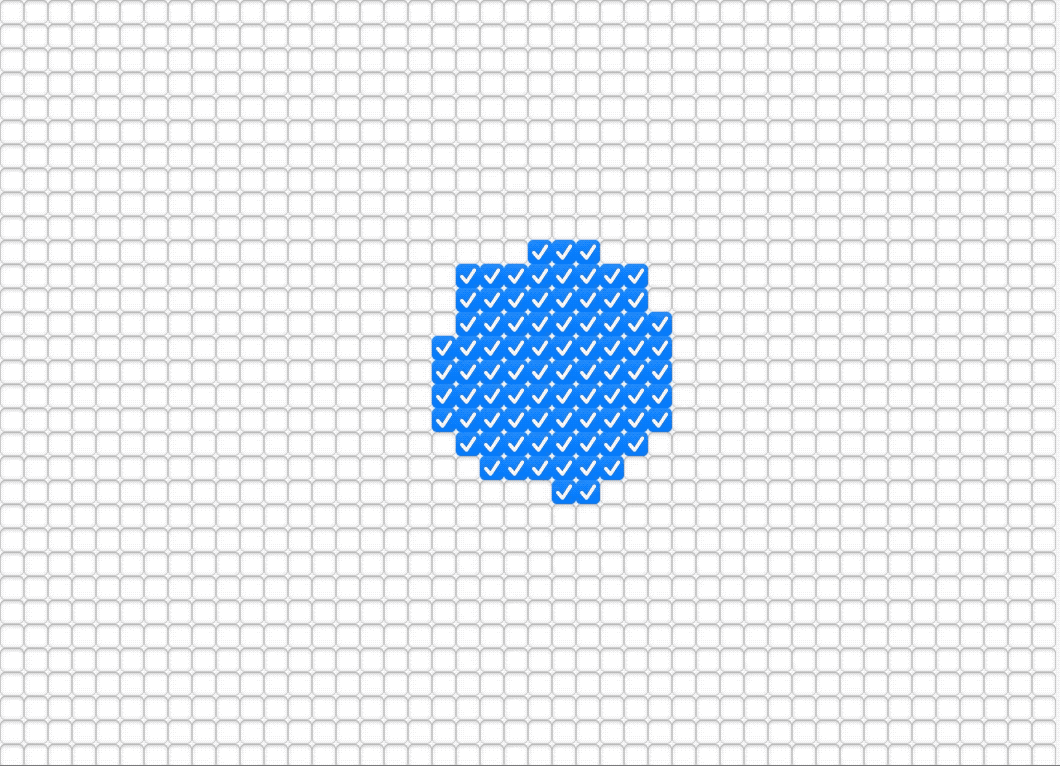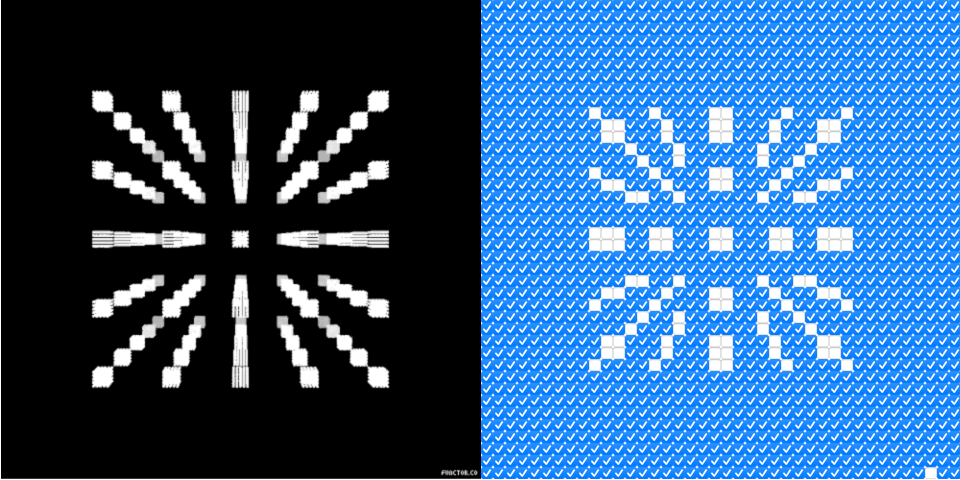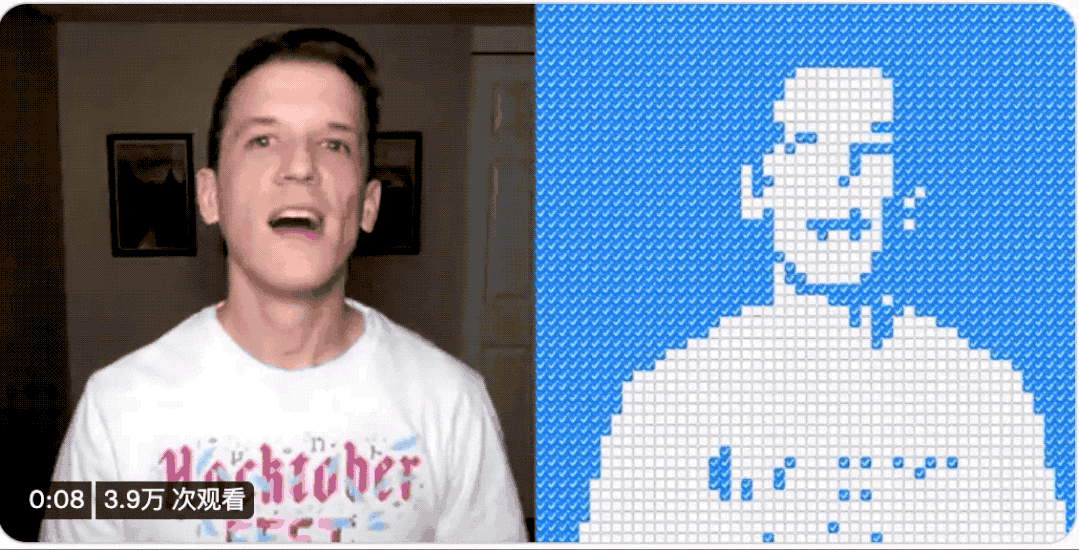1.做出原本的图像。

2.将图像转化为ASCII文本输出。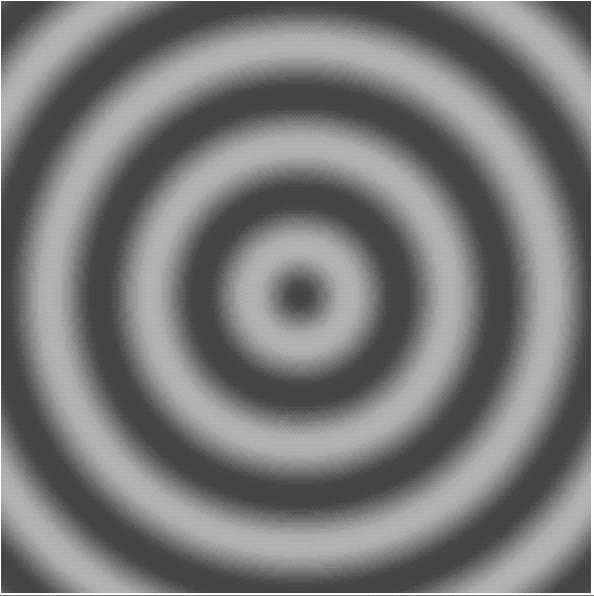z轴垂直屏幕向外，把z轴的数值转化为灰度，白色为波峰，黑色为波谷。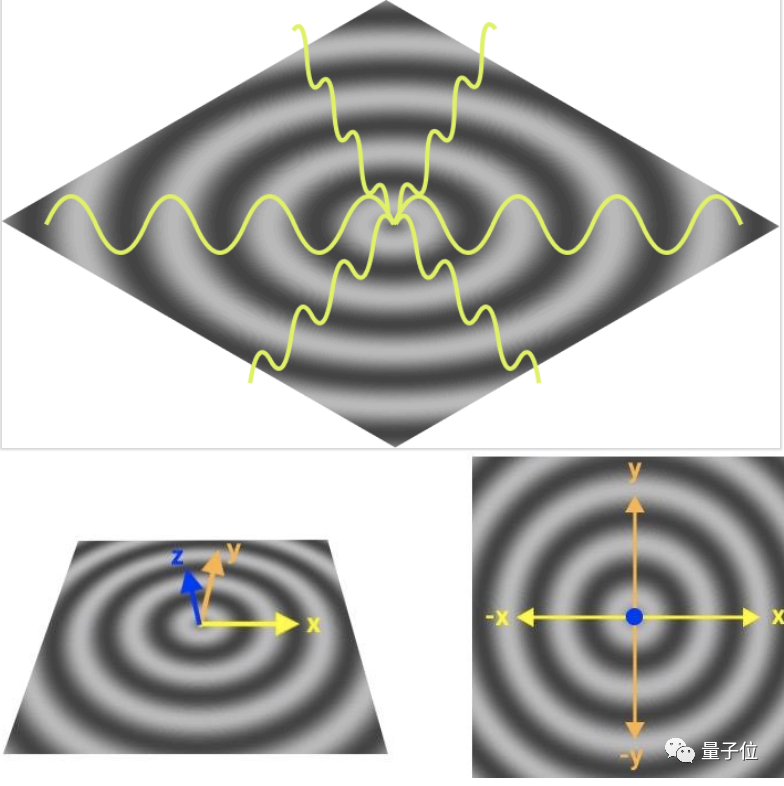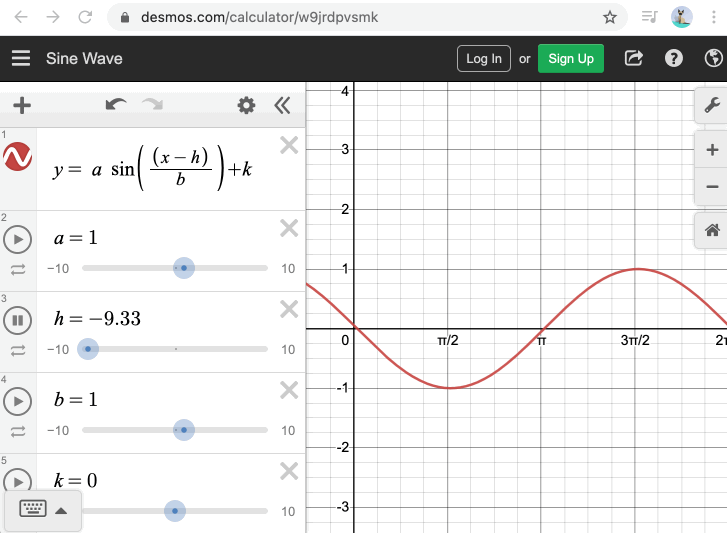`GrayScale = 0.21 R + 0.72 G + 0.07 B`

`const toGrayScale = (r, g, b) => 0.21 * r + 0.72 * g + 0.07 * b;`

`const convertToGrayScales = (context, width, height) => {`

`const imageData = context.getImageData(0, 0, width, height);`

`const grayScales = [];`

`for (let i = 0 ; i < imageData.data.length ; i += 4) {`

`const r = imageData.data[i];`

`const g = imageData.data[i + 1];`

`const b = imageData.data[i + 2];`

`const grayScale = toGrayScale(r, g, b);`

`imageData.data[i] = imageData.data[i + 1] = imageData.data[i + 2] = grayScale;`

`grayScales.push(grayScale);`

`}`

`context.putImageData(imageData, 0, 0);`

`return grayScales;`

`};`

`const grayRamp = '\$@B%8&WM#*oahkbdpqwmZO0QLCJUYXzcvunxrjft/|()1{}[]?-_+~<>i!lI;:,"^`\'. ';`

`const rampLength = grayRamp.length;`

`const getCharacterForGrayScale = grayScale => grayRamp[Math.ceil((rampLength - 1) * grayScale / 255)];`

`const asciiImage = document.querySelector('pre#ascii');`

`const drawAscii = (grayScales) => {`

`const ascii = grayScales.reduce((asciiImage, grayScale) => {`

`return asciiImage + getCharacterForGrayScale(grayScale);`

`}, '');`

`asciiImage.textContent = ascii;`

`};`